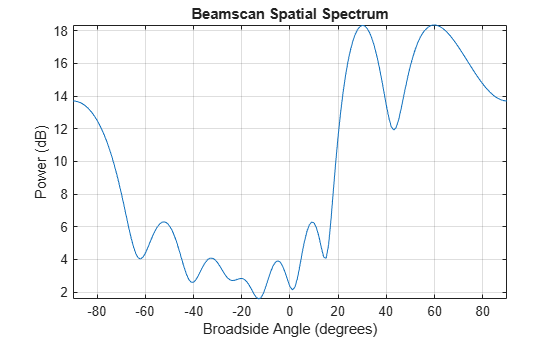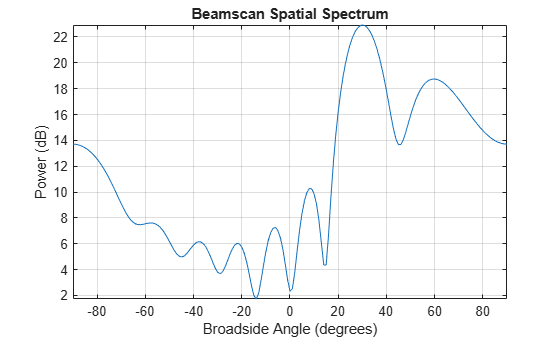Documentation

# sensorsig

Simulate received signal at sensor array

## Syntax

``x = sensorsig(pos,ns,ang)``
``x = sensorsig(pos,ns,ang,ncov)``
``x = sensorsig(pos,ns,ang,ncov,scov)``
``x = sensorsig(pos,ns,ang,ncov,scov,'Taper',taper)``
``````[x,rt] = sensorsig(___)``````
``````[x,rt,r] = sensorsig(___)``````

## Description

````x = sensorsig(pos,ns,ang)` simulates the received narrowband plane wave signals at a sensor array. `pos` represents the positions of the array elements, each of which is assumed to be isotropic. `ns` indicates the number of snapshots of the simulated signal. `ang` represents the incoming directions of each plane wave signal. The plane wave signals are assumed to be constant-modulus signals with random phases.```

example

````x = sensorsig(pos,ns,ang,ncov)` describes the noise across all sensor elements. `ncov` specifies the noise power or covariance matrix. The noise is a Gaussian distributed signal.```

example

````x = sensorsig(pos,ns,ang,ncov,scov)` specifies the power or covariance matrix for the incoming signals.```
````x = sensorsig(pos,ns,ang,ncov,scov,'Taper',taper)` specifies the array taper as a comma-separated pair consisting of `'Taper'` and a scalar or column vector.```
``````[x,rt] = sensorsig(___)``` also returns the theoretical covariance matrix of the received signal, using any of the input arguments in the previous syntaxes.```

example

``````[x,rt,r] = sensorsig(___)``` also returns the sample covariance matrix of the received signal.```

## Examples

collapse all

Simulate the received signal at an array, and use the data to estimate the arrival directions.

Note: This example runs only in R2016b or later. If you are using an earlier release, replace each call to the function with the equivalent `step` syntax. For example, replace `myObject(x)` with `step(myObject,x)`.

Create an 8-element uniform linear array whose elements are spaced half a wavelength apart.

```fc = 3e8; c = 3e8; lambda = c/fc; array = phased.ULA(8,lambda/2);```

Simulate 100 snapshots of the received signal at the array. Assume there are two signals, coming from azimuth 30° and 60°, respectively. The noise is white across all array elements, and the SNR is 10 dB.

```x = sensorsig(getElementPosition(array)/lambda,... 100,[30 60],db2pow(-10));```

Use a beamscan spatial spectrum estimator to estimate the arrival directions, based on the simulated data.

```estimator = phased.BeamscanEstimator('SensorArray',array,... 'PropagationSpeed',c,'OperatingFrequency',fc,... 'DOAOutputPort',true,'NumSignals',2); [~,ang_est] = estimator(x);```

Plot the spatial spectrum resulting from the estimation process.

`plotSpectrum(estimator)`The plot shows peaks at 30° and 60°.

Simulate receiving two uncorrelated incoming signals that have different power levels. A vector named `scov` stores the power levels.

Create an 8-element uniform linear array whose elements are spaced half a wavelength apart.

```fc = 3e8; c = 3e8; lambda = c/fc; ha = phased.ULA(8,lambda/2);```

Simulate 100 snapshots of the received signal at the array. Assume that one incoming signal originates from 30 degrees azimuth and has a power of 3 W. A second incoming signal originates from 60 degrees azimuth and has a power of 1 W. The two signals are not correlated with each other. The noise is white across all array elements, and the SNR is 10 dB.

```ang = [30 60]; scov = [3 1]; x = sensorsig(getElementPosition(ha)/lambda,... 100,ang,db2pow(-10),scov);```

Use a beamscan spatial spectrum estimator to estimate the arrival directions, based on the simulated data.

```hdoa = phased.BeamscanEstimator('SensorArray',ha,... 'PropagationSpeed',c,'OperatingFrequency',fc,... 'DOAOutputPort',true,'NumSignals',2); [~,ang_est] = step(hdoa,x);```

Plot the spatial spectrum resulting from the estimation process.

`plotSpectrum(hdoa);`The plot shows a high peak at 30 degrees and a lower peak at 60 degrees.

Simulate the reception of three signals, two of which are correlated.

Create a signal covariance matrix in which the first and third of three signals are correlated with each other.

```scov = [1 0 0.6;... 0 2 0;... 0.6 0 1];```

Simulate receiving 100 snapshots of three incoming signals from 30°, 40°, and 60° azimuth, respectively. The array that receives the signals is an 8-element uniform linear array whose elements are spaced one-half wavelength apart. The noise is white across all array elements, and the SNR is 10 dB.

```pos = (0:7)*0.5; ns = 100; ang = [30 40 60]; ncov = db2pow(-10); x = sensorsig(pos,ns,ang,ncov,scov);```

Simulate receiving a signal at a URA. Compare the signal theoretical covariance with its sample covariance.

Create a 2-by-2 uniform rectangular array having elements spaced 1/4-wavelength apart.

`pos = 0.25 * [0 0 0 0; -1 1 -1 1; -1 -1 1 1];`

Define the noise power independently for each of the four array elements. Each entry in `ncov` is the noise power of an array element. This element position is the corresponding column in `pos`. Assume the noise is uncorrelated across elements.

`ncov = db2pow([-9 -10 -10 -11]);`

Simulate 100 snapshots of the received signal at the array, and store the theoretical and empirical covariance matrices. Assume that one incoming signal originates from 30° azimuth and 10° elevation. A second incoming signal originates from 50° azimuth and 0° elevation. The signals have a power of 1 W and are uncorrelated.

```ns = 100; ang1 = [30; 10]; ang2 = [50; 0]; ang = [ang1, ang2]; rng default [x,rt,r] = sensorsig(pos,ns,ang,ncov);```

View the magnitudes of the theoretical covariance and sample covariance.

`abs(rt)`
```ans = 4×4 2.1259 1.8181 1.9261 1.9754 1.8181 2.1000 1.5263 1.9261 1.9261 1.5263 2.1000 1.8181 1.9754 1.9261 1.8181 2.0794 ```
`abs(r)`
```ans = 4×4 2.2107 1.7961 2.0205 1.9813 1.7961 1.9858 1.5163 1.8384 2.0205 1.5163 2.1762 1.8072 1.9813 1.8384 1.8072 2.0000 ```

Simulate receiving a signal at a ULA, where the noise between different sensors is correlated.

Create a 4-element uniform linear array whose elements are spaced one-half wavelength apart.

`pos = 0.5 * (0:3);`

Define the noise covariance matrix. The value in the ( k,_j_) position in the `ncov` matrix is the covariance between the k and j array elements listed in array.

`ncov = 0.1 * [1 0.1 0 0; 0.1 1 0.1 0; 0 0.1 1 0.1; 0 0 0.1 1];`

Simulate 100 snapshots of the received signal at the array. Assume that one incoming signal originates from 60° azimuth.

```ns = 100; ang = 60; [x,rt,r] = sensorsig(pos,ns,ang,ncov);```

View the theoretical and sample covariance matrices for the received signal.

`rt,r`
```rt = 4×4 complex 1.1000 + 0.0000i -0.9027 - 0.4086i 0.6661 + 0.7458i -0.3033 - 0.9529i -0.9027 + 0.4086i 1.1000 + 0.0000i -0.9027 - 0.4086i 0.6661 + 0.7458i 0.6661 - 0.7458i -0.9027 + 0.4086i 1.1000 + 0.0000i -0.9027 - 0.4086i -0.3033 + 0.9529i 0.6661 - 0.7458i -0.9027 + 0.4086i 1.1000 + 0.0000i ```
```r = 4×4 complex 1.1059 + 0.0000i -0.8681 - 0.4116i 0.6550 + 0.7017i -0.3151 - 0.9363i -0.8681 + 0.4116i 1.0037 + 0.0000i -0.8458 - 0.3456i 0.6578 + 0.6750i 0.6550 - 0.7017i -0.8458 + 0.3456i 1.0260 + 0.0000i -0.8775 - 0.3753i -0.3151 + 0.9363i 0.6578 - 0.6750i -0.8775 + 0.3753i 1.0606 + 0.0000i ```

## Input Arguments

collapse all

Positions of elements in sensor array, specified as an N-column vector or matrix. The values in the matrix are in units of signal wavelength. For example, `[0 1 2]` describes three elements that are spaced one signal wavelength apart. N is the number of elements in the array.

Dimensions of `pos`:

• For a linear array along the y axis, specify the y coordinates of the elements in a 1-by-N vector.

• For a planar array in the yz plane, specify the y and z coordinates of the elements in columns of a 2-by-N matrix.

• For an array of arbitrary shape, specify the x, y, and z coordinates of the elements in columns of a 3-by-N matrix.

Data Types: `double`

Number of snapshots of simulated signal, specified as a positive integer scalar. The function returns this number of samples per array element.

Data Types: `double`

Directions of incoming plane wave signals, specified as an M-column vector or matrix in degrees. M is the number of incoming signals.

Dimensions of `ang`:

• If `ang` is a 2-by-M matrix, each column specifies a direction. Each column is in the form ```[azimuth; elevation]```. The azimuth angle must be between –180 and 180 degrees, inclusive. The elevation angle must be between –90 and 90 degrees, inclusive.

• If `ang` is a 1-by-M vector, each entry specifies an azimuth angle. In this case, the corresponding elevation angle is assumed to be 0.

Data Types: `double`

Noise characteristics, specified as a nonnegative scalar, 1-by-N vector of positive numbers, or N-by-N positive definite matrix.

Dimensions of `ncov`:

• If `ncov` is a scalar, it represents the noise power of the white noise across all receiving sensor elements, in watts. In particular, a value of `0` indicates that there is no noise.

• If `ncov` is a 1-by-N vector, each entry represents the noise power of one of the sensor elements, in watts. The noise is uncorrelated across sensors.

• If `ncov` is an N-by-N matrix, it represents the covariance matrix for the noise across all sensor elements.

Data Types: `double`

Incoming signal characteristics, specified as a positive scalar, 1-by-M vector of positive numbers, or M-by-M positive semidefinite matrix.

Dimensions of `scov`:

• If `scov` is a scalar, it represents the power of all incoming signals, in watts. In this case, all incoming signals are uncorrelated and share the same power level.

• If `scov` is a 1-by-M vector, each entry represents the power of one of the incoming signals, in watts. In this case, all incoming signals are uncorrelated with each other.

• If `scov` is an M-by-M matrix, it represents the covariance matrix for all incoming signals. The matrix describes the correlation among the incoming signals. In this case, `scov` can be real or complex.

Data Types: `double`

Array element taper, specified as a scalar or complex-valued N-by-1 column vector. The dimension N is the number of array elements. If `taper` is a scalar, all elements in the array use the same value. If `taper` is a vector, each entry specifies the taper applied to the corresponding array element.

Data Types: `double`
Complex Number Support: Yes

## Output Arguments

collapse all

Received signal at sensor array, returned as a complex `ns`-by-N matrix. Each column represents the received signal at the corresponding element of the array. Each row represents a snapshot.

Theoretical covariance matrix of the received signal, returned as a complex N-by-N matrix.

Sample covariance matrix of the received signal, returned as a complex N-by-N matrix. N is the number of array elements. The function derives this matrix from `x`.

### Note

If you specify this output argument, consider making `ns` greater than or equal to N. Otherwise, `r` is rank deficient.

## More About

collapse all

### Azimuth Angle, Elevation Angle

The azimuth angle of a vector is the angle between the x-axis and the orthogonal projection of the vector onto the xy plane. The angle is positive in going from the x axis toward the y axis. Azimuth angles lie between –180 and 180 degrees. The elevation angle is the angle between the vector and its orthogonal projection onto the xy-plane. The angle is positive when going toward the positive z-axis from the xy plane. These definitions assume the boresight direction is the positive x-axis.

### Note

The elevation angle is sometimes defined in the literature as the angle a vector makes with the positive z-axis. The MATLAB® and Phased Array System Toolbox™ products do not use this definition.

This figure illustrates the azimuth angle and elevation angle for a vector that appears as a green solid line. The coordinate system is relative to the center of a uniform linear array, whose elements appear as blue circles.## See Also

#### Hybrid Beamforming for Massive MIMO Phased Array Systems

Download the white paper